## Thursday, November 18, 2010

### Beamcasting

I am Topi "Sharph" Talvitie, the other coder in the Umbrella Project. I've been mostly helping with path finding related algorithms in the project.

Efficient path finding algorithms run on a graph of links between vertices. These graphs are a bit large to be distributed as precalculated data, so they need to be generated at runtime. If possible, this process should be fast enough to be unnoticeable. Our solution to the problem is casting a beam from each vertex to find the vertices that this vertex is linked to.

In the figure below there is a beam (the red area) cast from vertex A of polygon P. The vertices seen from vertex A are circled.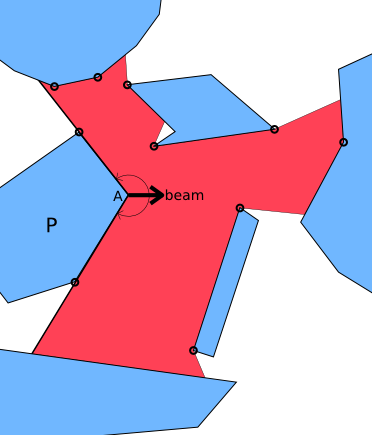If we are able to query the whole visible polygon from any point, we can use the same algorithm for many interesting effects too, such as checking if the player is seen by a character in the game. Thus we need an algorithm that can cast a beam from both polygon vertices and free space.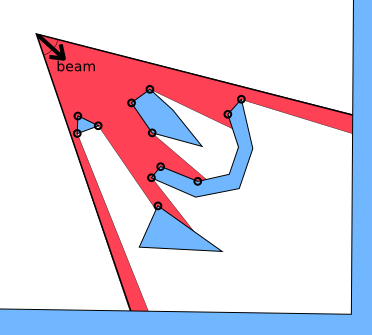So how to do this efficiently? After considering some grid accelerated algorithms that turned out to be quite complex, we realized that a triangle tessellation (triangulation) of the map would be very useful.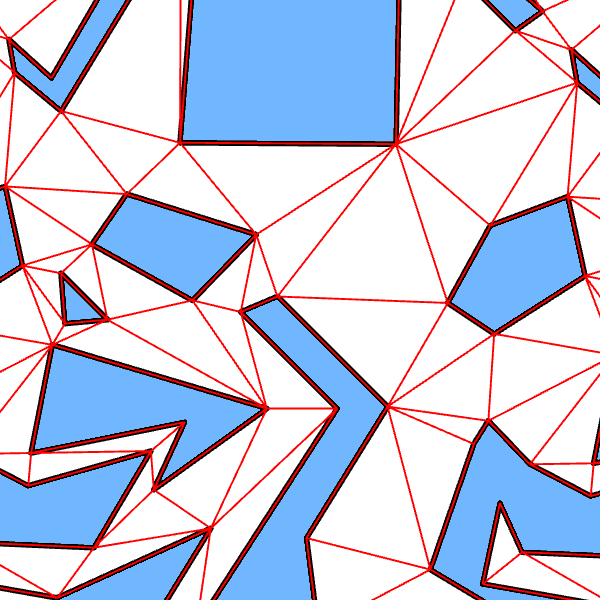We will shortly describe the algorithm. There's still a cake for you in the action phase after that.

### Initiating the Cast

Because the polygon map is tessellated, the starting point of the beam O (lower right) is an interior point of exactly one triangle T. We divide the beam (lower left) into at most three smaller beams (lower right: A, B and C) by splitting it by the sectors to the triangle edges.

Each of these smaller beams sees exactly one edge of the triangle T. If that edge is a wall of a polygon, just add it. For the unblocked beams, we advance to the next neighboring triangle. (N1 for A, N3 for B, N2 for C)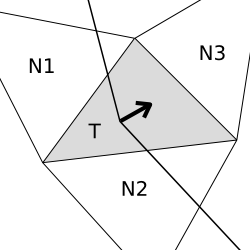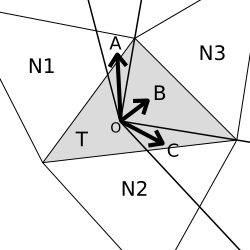The case of starting from a vertex is very similar.

### Recursion

In the recursion step we consider a smaller beam, recursed to a triangle (the lower triangle in the figures below). Due to splitting the beam when entering new triangles, the beam will enter the neighboring triangle (gray) through exactly one edge (L-R).

We take the line to the opposite vertex of this new triangle (lower right, O-S) and use this line to deduce which neighbors of the current triangle (gray) will need to be recursed into. If both edges (L-S, S-R) are seen, both neighboring triangles will need to be analyzed (lower right).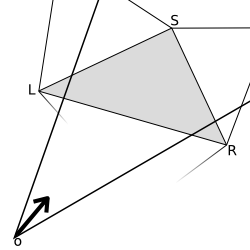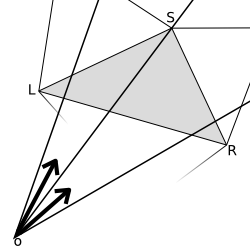If only the leftmost edge is seen, only the left triangle will need to be analyzed (lower left). If only the rightmost edge is seen, only the right triangle will need to be analyzed.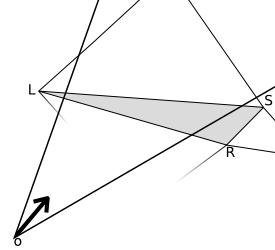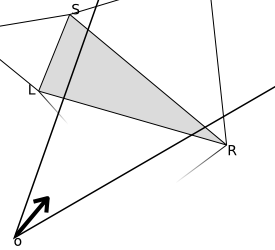Again, if the triangle we are advancing into is part of an obstacle polygon, just add the edge. And finally, to keep the result polygon correctly ordered, the search should be done depth-first and always to the same direction first.

There exist many good libraries for triangulation. We used poly2tri, licensed under the New BSD License.

### Something to think...

It is proven that in pathfinding, links from vertex A to vertices in beam C are not needed. Can you figure out why? Might be confusing at first!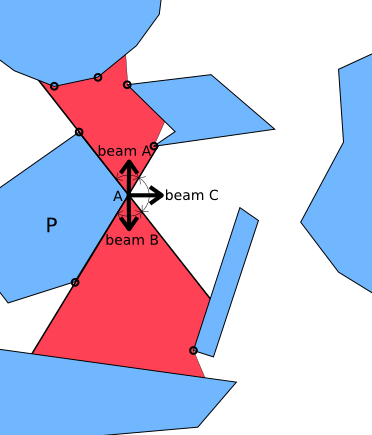And at last...

### Action!

We prepared a scene for your investigation for this very realistic simulation of a
crime-in-action, a western style bank robbery. The blue casting star represents the sheriff and the red casting robber represents the bad guy. Enjoy!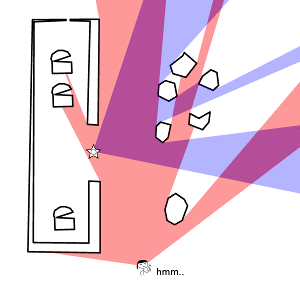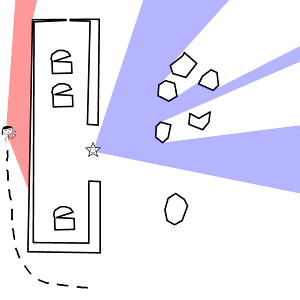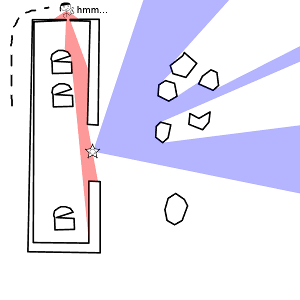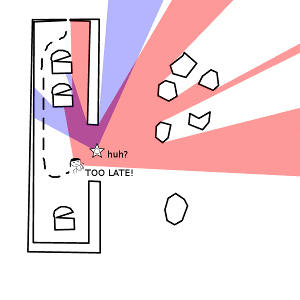(Written by Topi Talvitie, edited by Markus Kettunen)